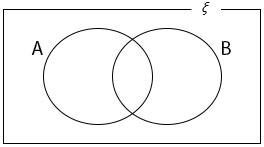### Set Operations

Mathematics SSS 1 First Term

WEEK 10

Set Operations

Performance Objectives

Students should be able to;

1. Carry out all set operations
2. Draw Venn diagram
3. Interpret Venn diagrams and use Venn diagrams

Union of Sets

Content

The Union of Sets A and B is the Set that is formed from the elements of the two sets A and B. This is usually denoted by “A B” meaning A Union B. Thus A B is the Set which consists of elements of A or B or both A and B.

When represented using Venn diagram we have
A
BView More